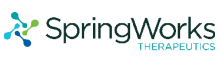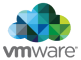New update is available. Click here to update.
Last Updated: 4 Nov, 2020

# Equilibrium indices of a Sequence

Moderate+1 more companies

## Problem statement#### The equilibrium index of a sequence of integers is defined as the index such that the sum of all the elements at lower indices is equal to the sum of elements at higher indices.

##### Note:
``````1. 'SEQUENCE' may contain more than one equilibrium indices.

2. If there are no equilibrium indices, return an empty sequence.

3. Consider the sum of elements lower than the first index and higher than the last index to be 0 (zero).
``````
##### Input format:
``````The first line of input contains an integer ‘T’ denoting the number of test cases.

The first line of each test case contains an integer ‘N’ denoting the number of elements in the given array/list 'SEQUENCE'.

The second line of each test case contains ‘N’ space-separated integers denoting the elements in the 'SEQUENCE'.
``````
##### Output Format:
``````For each test case, return a sequence of equilibrium indices. If no such index exists, return an empty sequence.
``````
##### Note:
``````You don't need to print the output, it has already been taken care of. Just implement the given function.
``````
##### Constraints:
``````1 <= T <= 50
1 <= N <= 10^4
-10^9 <= SEQUENCE[i] <= 10^9

Where 'SEQUENCE[i]' denotes the elements of the array 'SEQUENCE'.

Time limit: 1 sec
``````## Approaches

### 01 ApproachFor an index to be at equilibrium we need to calculate the sum of all the elements present at indices lower than the index and the sum of all the elements present at indices higher than the index. If both the sums match then we can add that index to the sequence of equilibrium indices. Hence, for a given sequence we can iterate through each of the indexes and store it as ‘currentIndex’.

Now iterate through all the elements present at indices lower than ‘currentIndex’ and store it as ‘lowerSum’. Similarly, iterate through all the elements present at indices higher than ‘currentIndex’ and store it as ‘higherSum’. If ‘lowerSum’ matches ‘higherSum’ than the ‘currentIndex’ is at equilibrium and we add it to the answer sequence, else we traverse further in the sequence.

Once we complete the traversal we can return the answer sequence, which contains all the equilibrium indices or empty sequence in case of no equilibrium indices.